﻿ Numerical Method for the Solution of Logistic Differential Equations of Fractional OrderPublications are Open
Access in this journal
Article Versions
Export Article
• Normal Style
• MLA Style
• APA Style
• Chicago Style
Research Article
Open Access Peer-reviewed

Numerical Method for the Solution of Logistic Differential Equations of Fractional Order

Hatıra Günerhan
Turkish Journal of Analysis and Number Theory. 2019, 7(2), 33-36. DOI: 10.12691/tjant-7-2-1
Received February 02, 2019; Revised March 08, 2019; Accepted March 15, 2019

Abstract

In this paper, Numerical solution of Fractional Order Logistic Equations is considered by fractional differential transform method (FDTM). The method provides the solution quickly in the form of a convergent series. Our method reduces the computational difficulties existing in the other traditional methods. To show the efficacy of the results, two examples are considered.

1. Introduction

In recent years, fractional modeling and fractional differential equations has been used widely to deal with some engineering and industrial problems. The applications of fractional calculus can be studied in many scientific disciplines based on mathematical modeling including physics, chemistry, aerodynamics, signal processing, electrodynamics, economics, biophysics, polymer rheology, blood flow phenomena, control theory and many others. For this reason this area caught the interest of many researchers recently. Fractional derivative is a powerful tool which has been recently employed to model complex biological systems with non-linear behavior and long-term memory 1, 2, 3, 4, 5, 6, 7. Most nonlinear fractional differential equations may not be solved analytically, alternatively approximations and numerical approaches should be used for them.

The fractional Logistic model can be obtained by applying the fractional derivative operator to the Logistic equation. As known, Pierre F. Verhulst introduced the nonlinear term into the rate equation and obtained what today is known as the logistic equation 8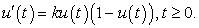Here we consider the fractional order version of the standard logistic equation as 9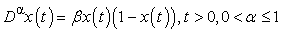with an initial condition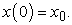Fractional order logistic equation (FOLE) has no known exact solution yet 10, 11, 12. So we study on the numerical solution of the equation.

The DTM was firstly proposed by Zhou 13 for solving linear and nonlinear initial value problems in electric circuit. The DTM is numerical method based on Taylor series expansion, which constitute a polynomial form of analytic solution. So indeed the DTM obtains a polynomial series solution by means of an iterative procedure 14. DTM is used in many studies related to differential or partial differential equations 15, 16, 17, 18, 19, 20, 21, 22.

The differential transform method is an analytic method based on the Taylor series expansion which constructs an analytical solution in the form of a polynomial. The traditional high order Taylor series method requires symbolic computation. However, the FDTM obtains a polynomial series solution by means of an iterative procedure. The proposed method is based on the combination of the classical one dimensional FDTM and generalized Taylor’s Table 1 formula.

2. Basic Definitions

Definition 1. A real function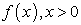is said to be in the space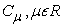if there exists a real number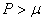such that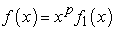where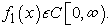Clearly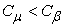if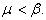Definition 2. A functionis said to be in the space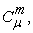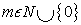if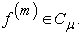Several definitions have been made to define the fractional derivative and continuous to be done. Most popular definitions in this area are the Riemann-Liouville and Caputo.

Definition 3. The Riemann-Liouville fractional integral operator of order a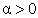of a function,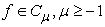is defined as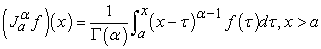(1)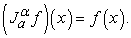(2)

Properties of the operator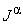can be found in (Caputo, 1967), we mention only the following:

For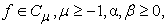and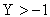a)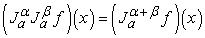(3)

b)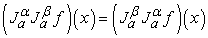(4)

c)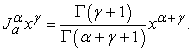(5)

The Riemann–Liouville derivative has certain disadvantages when trying to model real world phenomena with fractional differential equations. Therefore, we shall introduce a modified fractional differential operator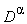proposed by Caputo (1967) in his work on the theory of viscoelasticity.

Definition 4. The fractional derivative of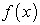in the Caputo sense is defined as(6)

For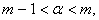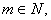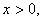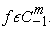Lemma 1. If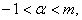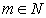and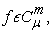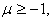then

a)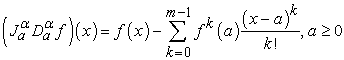(7)

b)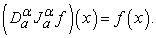(8)

3. Fractional Differential Transform Method

The fractional differentiation in Riemann–Liouville sense is defined see 23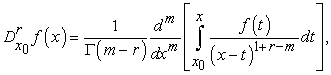(9)

for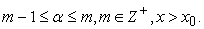Let us expand the analytical and continuous function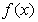in terms of a fractional power series as follows: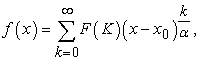(10)

where α is the order of fraction and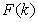is the fractional differential transform of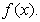In order to avoid fractional initial and boundary conditions, we define the fractional derivative in the Caputo sense. The relation between the Riemann–Liouville operator and Caputo operator is given by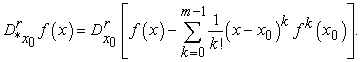(11)

Substituting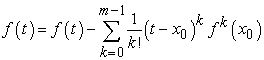in Eq. (9), we obtain fractional derivative in the Caputo sense as: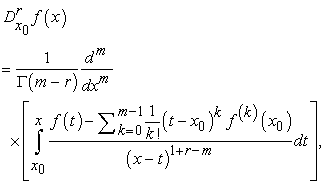(12)

the transformation of the initial conditions are defined as follows: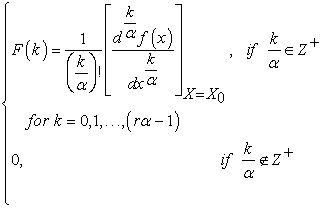Some basic properties of the fractional differential transform method are introduced in Table 1.

4. Numerical Example

Here, fractional differential transform method (FDTM) will be applied for solving Fractional Order Logistic Equations. The results reveal that this method is very effective.

Example 1. Consider the Fractional Order Logistic Equations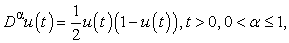(13)

with initial condition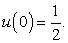(14)

Applying the FDTM to Eq. (13), the following recurrence relation can be obtained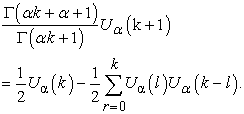(15)

From the initial condition given by Equation (14), we obtained: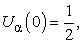(16)

the values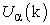in k = 0,1,2,… of equation (15) and (16) can be evaluated as follows: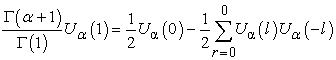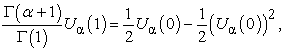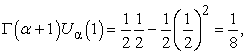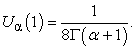Similarly, we have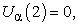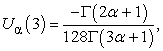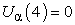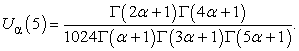(17)

By using the differential inverse reduced transform of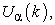we get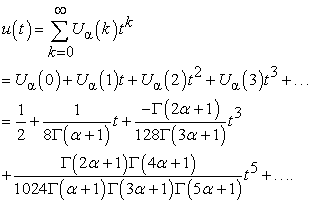(18)

By substituting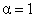into Equation (18), we have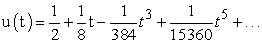the closed form solution will be as follows: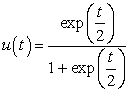(19)

which is exact solution.

Example 2. Consider the Fractional Order Logistic Equations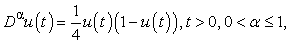(20)

with initial condition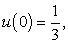(21)

by using the basic properties of RDTM in equation (20) and (21), we obtain the following relations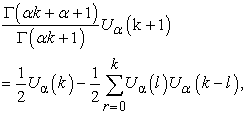(22)

from the initial condition given by Equation (21), we obtained: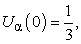(23)

the valuesin k = 0,1,2,… of equation (22) and (23) can be evaluated as follows: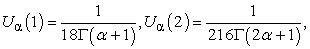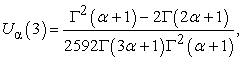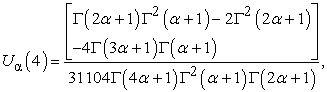(24)

by using the differential inverse reduced transform of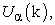we get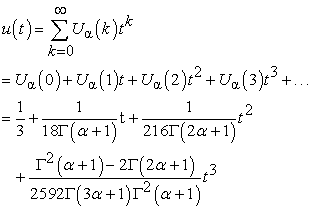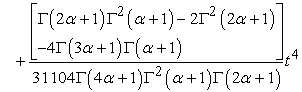(25)

by substitutinginto Equation (25), we have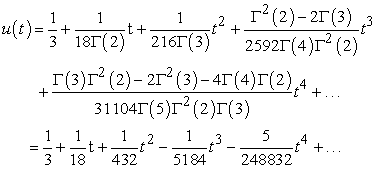(26)

The closed form solution will be as follows: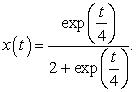(27)

5. Conclusion

In this paper, the fractional differential transform method (FDTM) has been utilized to solve fractional order differential equation. The results obtained by the method are in good compatible with the given exact solutions for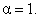The study show that the method is effective and suitable techniques to solve fractional order logistic equations. On the other hand the results are quite reliable for solving this problem.

References

  R. Hilfer, Applications of Fractional Calculus in Physics. World Scientific, Singapore, 2000. In article View Article  PubMed  F. Mainardi, Fractional calculus: Some basic problems in continuum and statistical mechanics, in: A. Carpinteri, F. Mainardi (Eds.), Fractals and Fractional Calculus in Continuum Mechanics, Springer, New York, 291-348. 1997. In article View Article  A. Carpinteri, F Mainardi. Fractals and Fractional Calculus in Continuum Mechanics, Springer Verlag, Wien, New York, 1997. In article View Article  PubMed  A.B. Malinowska, D.F.M. Torres, “Towards a combined fractional mechanics and quantization,” Fractional Calculus and Applied Analysis 15(3). 407-417. 2012. In article View Article  B. İbiş. M. Bayram. “Numerical comparison of methods for solving fractional differential-algebraic equations (FDAEs),” Computers and Mathematics with Applications 62. 3270-3278. 2011. In article View Article  H. Jafari, K. Sayevand, H. Tajadodi, D. Baleanu, “Homotopy analysis method for solving Abel differential equation of fractional order”, Central European Journal of Physics, 11 (10). 1523-1527. 2013. In article View Article  I. Podlubny. Fractional Differential Equations. An Introduction to Fractional Derivatives Fractional Differential Equations Some Methods of their Solution and Some of their Applications, Academic Press, San Diego, 1999. In article  P.F.Verhulst, “Notice sur la loi que la population sint dons son accroissement,” Math. Phys.10. 113. 1838. In article  S. Bhalekar, V. D. Gejji, “Solving fractional order Logistic equation using a new Iterative method”, International Journal of Differential Equations, 2012. 12. 2012. In article View Article  H. Khan, M. Alipour, R. Ali Khan, H. Tajadodi, A. Khan. “On Approximate Solution Of Fractional Order Logistic Equations By Operational Matrices Of Bernstein Polynomials,“ Journal of Mathematics and Computer Science. 14. 222-232. 2015. In article View Article  U. Forys, A. Marciniak-Czochra, “Logistic equations in tumor growth modelling,” Int. J.Appl. Math. Comput. Sci. 13. 317. 2003. In article  T.C. Fisher, R.H. Fry, “A simple substitution model of technological change, Technol. Forecast,” Soc. Change 3. 75. 1971. In article View Article  J.K. Zhou, Differential Transformation and its Application for Electrical Circutits, Huarjung University Press, Wuuhahn China, 1986. In article  B.Ibiş, M.Bayram and A. G. Ağargun, “Applications of conformable fractional differential transform method to Fractional Differential-Algebraic Equations,” Europan Journal of Pure and Applied Mathematics. 2. 129-141. 2011. In article  Ayaz, F., “Solutions of the system of differential equations by differential transform method,” Appl. Math. Comput. 147. 547-567. 2004. In article View Article  I.H. Abdel-Halim Hassan, “Application to differential transformation method for solving systems of differential equations,” Appl. Math. Modell. 32(12). 2552–2559. 2008. In article View Article  H. Liu, Yongzhong Song, “Differential transform method applied to high index differential algebraic equations,” Appl. Math. Comput. 184. 748-753. 2007. In article View Article  A. Arikoglu, I. Ozkol, “Solution of boundary value problems for integro-differential equations by using differential transform method,” Appl. Math. Comput. 168. 1145-1158. 2005. In article View Article  A. Arikoğlu, I. Ozkol. “Solution of fractional differential equations by using differential transform method.” Chaos, Solitons and Fractals. 34-5. 1473-1481. 2007. In article View Article  Adel Al-rabtah, Vedat Suat Ertürk, Shaher Momani, “Solutions of a fractional oscillator by using differential transform method,” Computers & Mathematics with Applications, 59(3). 1356-1362. 2010. In article View Article  Ertürk, Vedat Suat, Shaher Momani, “Solving systems of fractional differential equations using differential transform method,” J. Comput. Appl. Math. 215(1). 142-151. 2008. In article View Article  Secer, Aydin, Mehmet Ali Akinlar, Adem Cevikel, “Efficient solutions of systems of fractional PDEs by the differential transform method,” Adv. Difference Equ. 2012(1). 1-7. 2012. In article View Article  D. Nazari, S. Shahmorad, “Application of the Conformable fractional differential transform method to fractional-order integro-differential equations with nonlocal boundary conditions,” J. Comput. Appl. Math. 234 (3). 883-891. 2010. In article View ArticleThis work is licensed under a Creative Commons Attribution 4.0 International License. To view a copy of this license, visit http://creativecommons.org/licenses/by/4.0/